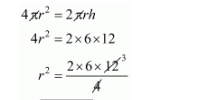# The surface area of a sphere is same as the curved`
Question:

The surface area of a sphere is same as the curved surface area of a right circular cylinder whose height and diameter are 12 cm each. The radius of the sphere is

(a) 3 cm

(b) 4 cm

(c) 6 cm

(d) 12 cm

Solution:

Let r be the radius of sphere

But,

Surface area of sphere = C.S.A. of cylinder$r=6 \mathrm{~cm}$

Hence, the correct answer is choice (c).